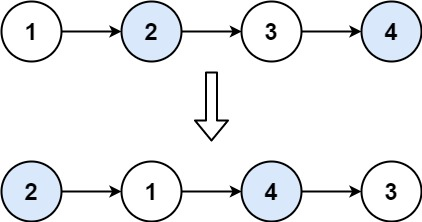# GeetCode Hub

Given a linked list, swap every two adjacent nodes and return its head. You must solve the problem without modifying the values in the list's nodes (i.e., only nodes themselves may be changed.)

Example 1:```Input: head = [1,2,3,4]
Output: [2,1,4,3]
```

Example 2:

```Input: head = []
Output: []
```

Example 3:

```Input: head = 
Output: 
```

Constraints:

• The number of nodes in the list is in the range `[0, 100]`.
• `0 <= Node.val <= 100`

/** * Definition for singly-linked list. * public class ListNode { * int val; * ListNode next; * ListNode() {} * ListNode(int val) { this.val = val; } * ListNode(int val, ListNode next) { this.val = val; this.next = next; } * } */ class Solution { public ListNode swapPairs(ListNode head) { if(head == null || head.next == null){ return head; } ListNode dummy = new ListNode(0); ListNode prev = dummy; while(head != null && head.next != null){ ListNode sec = head.next; head.next = sec.next; sec.next = head; prev.next = sec; prev = sec.next; head = head.next; } return dummy.next; } }

We can solve this question using both Recursive and Iterative approaches. I have discussed both solutions in detail in the Video. Once you know how to play with pointers, then this question will be easy to code.

Time Complexity: O(n)
Space Complexity: O(1)